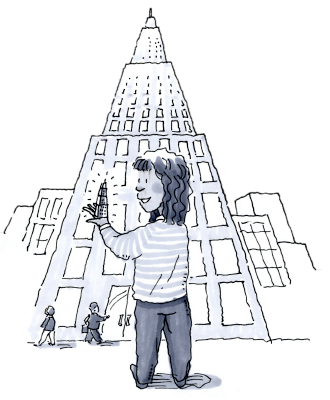### Home > MC1 > Chapter 7 > Lesson 7.2.1 > Problem7-35

7-35.

Glenda made a scale model of the Empire State Building in New York. The building itself is $1250$ feet high, and her scale model is $5$ feet high.

1. Write a ratio to compare the heights of the two buildings.

Choose one of the ways below.

$\frac{\text{scale model}}{\text{building}}$        $\frac{\text{building}}{\text{scale model}}$

2. If the entry of Glenda's model is $2$ inches wide, how wide would you expect the entry of the real building to be?

$41$ feet, $8$ inches. Remember to show your work.

3. If the real building contains $102$ floors, how many floors should Glenda's model contain?

Do you change the number of floors or the size of each floor?